### Choose language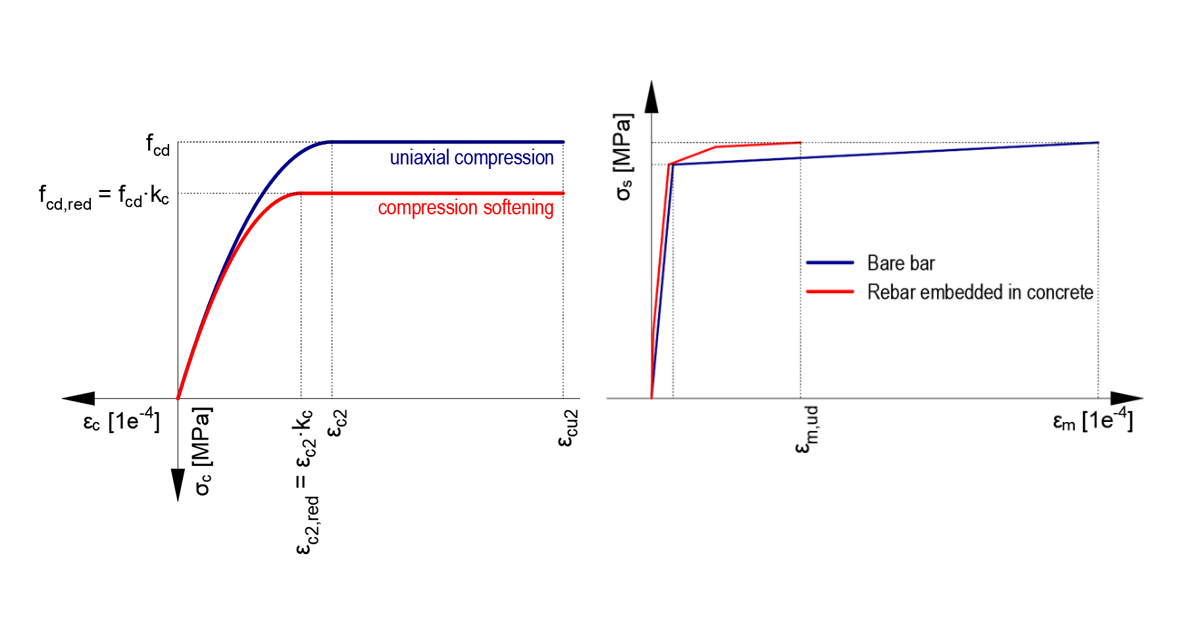# Stress-strain diagrams in CSFM

$$In the first part of the article, we will focus on the stress-strain diagrams themselves:Stress-strain diagrams implemented in CSFM are based on code requirements, both Eurocode (EN) and ACI. When dealing with concrete, it is necessary to distinguish between ULS and SLS assessments. A concrete member in the ultimate limit state (ULS) is based on the parabolic-rectangular diagram (EN) or bilinear one (ACI), including the compression softening effect. For serviceability assessments, a different, simpler diagram is used, a linear or bi-linear diagram with an infinite elastic branch. Bilinear diagrams with an inclined or horizontal top branch are considered for reinforcement, including tension stiffening effects for both ULS and SLS checks.Check the video below, where all stress-strain diagrams are displayed and briefly described. A more detailed description of stress-strain relationships is explained in the theoretical background. Now when it is clear what diagrams are used. Let's see how the Elastic module E is calculated:ULSElastic modulus is calculated from concrete stress value at specific age (introduce concrete compressive strength growth with age) and strain value at maximal strength values.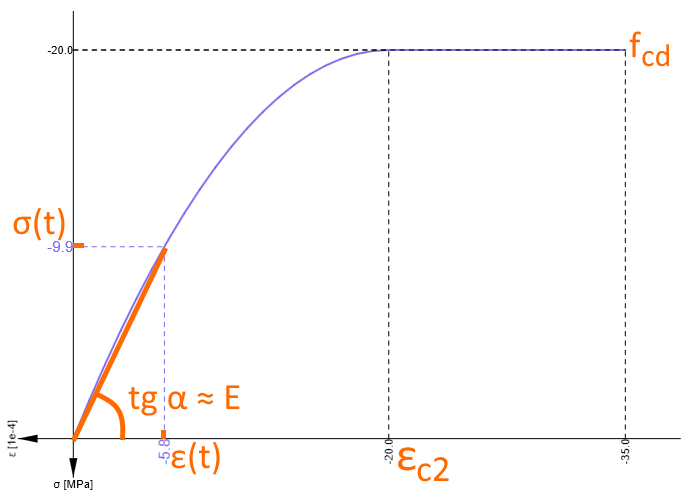The following table shows the growth of elastic modulus over time in the last column, where after the age of 28 days, the maximal value of 10GPa is reached.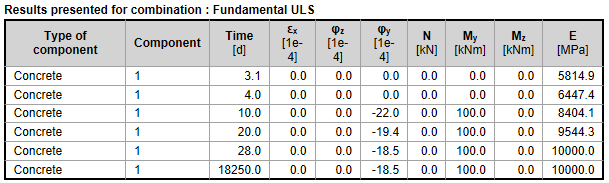SLSThe code considers Ecm value set for 0,4fcm as secant elastic modulus in chapter 3.1.3.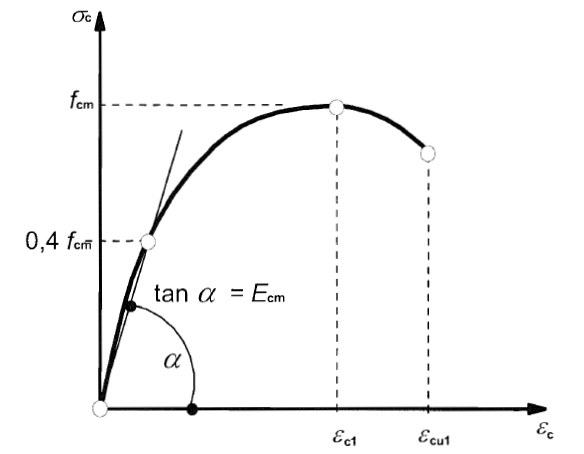In paragraph (3) it states: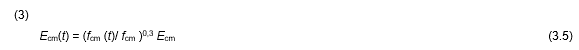The following table shows the growth of elastic modulus considered for characteristic combination (SLS):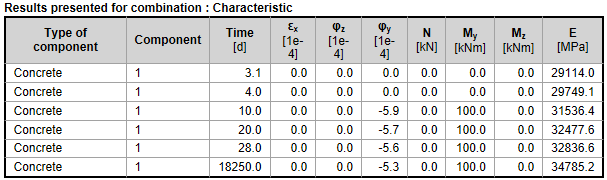The type of aggregate also changes the calculation of elastic modulus. The aggregate type used in concrete is set for Quarzite as a default value. The reduction of modulus is considered for limestone or sandstone aggregate, and for basalt aggregate, it applies the increase of elastic modulus.$$